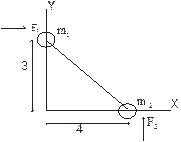# Objective type questions on linear momentum and system of particles for CBSE,JEE Advanced/Main, AP Physics

In this page we have Objective type questions on linear momentum and system of particles for CBSE,JEE Advanced/Main, AP Physics . Hope you like them and do not forget to like , social share and comment at the end of the page.
Question 1.
(i). Two masses constrained to move in a horizontal plane collide. Given initially that M 1 = 85gm and  M2 = 200gm, v1 = 6.4i cm/sec and v2 = -6.7i - 2j cm/sec.
i and j are the unit vectors across x and y axis respectively
Find the Velocity of Center of mass
(a) -2.8i - 1.4j
(b) 2.8i + 1.4j
(c) 2i + 1.4j
(d) none of the above
(ii). Find the total Linear Momentum of the system
(a) -798i - 400j
(b) -796i + 400j
(c) 600i + 400j
(d) none of the above
(iii). Find the velocity in the reference of frame in which centre of mass is at rest
(a) 9.2i - 1.4j ,- 3.9i - 0.6j
(b) 9.2i + 1.4j ,- 3.9i - 0.6j
(c) -9.2i + 1.4j, - 3.9i - 0.6j
(d) none of the above
(iv) Let w1 and w2 are final velocity
now we know |w1| = 9.2 and w2 = 4.4i + 1.9j cm/sec
find the direction of w1
(a) 26° with respect to x axis
(b) 84° with respect to x axis
(c) -84° with respect to x axis
(d) none of the above
Question 2.
A straight rod of length L has one of its end at origin  and other at (L, 0). If the mass per unit length of rod is  Ax + B .Find the centre of mass
(a) L(2AL + 3B)/(3AL + 6B)
(b) L(AL + 3B)/(AL + 6B)
(c) L(AL - B)/(AL + 2B)
(d) none of the above
Question 3.
A uniform solid sphere has a spherical hole in it. Find the centre of mass
(a) xcm = -a3b/(R3-a3), ycm = 0, zcm = 0
(b) xcm = a3b/(R3-a3), ycm = 0, zcm = 0
(c) xcm = b3a/(R3-a3), ycm = 0, zcm = 0
(d) none of the above

Question 4.
(i). Two mass m1= 10 and m2 = 6 are joined by a rigid bar of negligible mass as shown in FigureBeing initially at rest, they are subjected to force F1 = 8i N and F2 = 6j N
Find the coordinate of their centre of mass as a function of time
(a) i(1.5 - 0.25t2) + j(1.88 - 0.188t)
(b) i(1.5 + 0.25t2) + j(1.88 + 0.188t2)
(c) (1.5 + 0.188t2) i
(d) none of the above
(ii). Find the total linear momentum as function of time
(a) (8i + 6j)t
(b) (8i -6j)t
(c) (2i +6j)t
(d) none of the above
Question 5.
A man of mass m standing is on a block of mass M. The system is at rest. The man moves relative in x direction to the block with velocity v' and then stops.
Find the velocity of centre of mass
(a) v'
(b) 0
(c) mv'/M + m
(d) none of the above
Question 6.
Three bodies form an isolated system. There are m1 = m2 = 2m and m3 = 3m. They have different direction, but all have the same initial speed v0. One or more elastic collision between the pair of the bodies where otherwise do not intersect. Find the maximum possible final speed of each of the three bodies.
(a) 3v0, 2v0, v0
(b) v0, 2v0, 3v0
(c) 2.4v0, 1.73v0, 1.41v0
(d) none of the above
Question 7.
A ball is dropped from height h on a floor where Coefficient of restitution is e. Find the time required by the ball to stop rebounding
(a) √(2h/g) (1 + e/1 - e)
(b) √(2h/g) (1 + e)
(c) √(2h/g) (1 - e/1 + e)
(d) none of the above

Question 8. Matrix match type-
In a system of particle
Column A
(a) external force are present, but no internal force
(b) internal force are present, but no external force
(c) both no external and internal force
(d) both internal and external force are presen
Column B
(P) total momentum will remain constant
(Q) total momentum will not remain constant
(R) centre of mass will accelerate
(S) centre of mass will not accelerate
1. (i) – (a) : (ii) – (a) : (iii) – (b) : (iv) – (c)
2. (a)
3. (a)
4. (i) - (b) : (ii) – (a)
5. (b)
6. (c)
7. (a)
8. Matrix match type : (a) → (Q), (R) : (b) → (P), (S) : (c) → (P), (S) : (d) → (P), (S)

Solutions